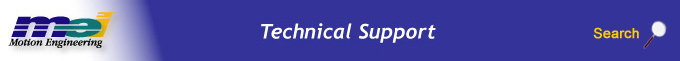.

Block Execution Order and User Defined Block Priority

## Block Execution Order

The MechaWare Model Downloader uses a simple algorithm to determine the block execution order. The block processing order is determined at download time. The blocks are listed in the order of processing in the associated .map file generated by the downloader.

For each block, calculate the ratio between the number of inputs to the block that have already been executed and the total number of inputs. Choose the block with the highest ratio. If two or more blocks have the highest ratio, choose the block whose latest input is scheduled the earliest. If the blocks are still equal, the blocks are calculated in the order they are stored in the model file by Simulink. With the current version of Simulink this is in alphabetical order by block name. Repeat until all blocks are scheduled.

Note: Alphabetically ordered blocks are sorted character-by-character, which can cause confusion. For example, a block named SUM 11 is processed before a block named SUM 2.

For models without loops, this guarantees that no block are executed before any of its inputs. For models with loops, it minimizes the number of single-cycle delays in the system.

For example, consider the following model: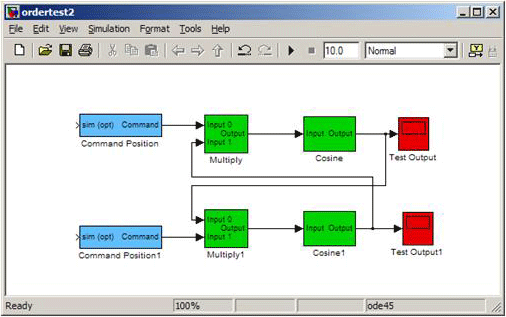Command Position and Command Position 1 are scheduled first, as both of them have 100% of their inputs computed. At this point, Multiply and Multiply 1 each have 50% of their inputs computed, while the remaining four blocks have none of their inputs computed. Because Multiply has the earliest scheduled input (Command Position), Multiply is scheduled next. Cosine has 100% of its inputs computed, so it is scheduled next. This process continues until all blocks are scheduled accordingly. The final ordering is:

1. Command Position
2. Command Position1
3. Multiply
4. Cosine
5. Multiply1
6. Test Output
7. Cosine1
8. Test Output1

User Defined Block Priority

If this algorithm is not producing the block ordering you want, you can use the User Defined Block Priority field on any block. If the Priority field is set, the block is guaranteed to be executed before any other blocks with a larger Priority value. Multiple blocks can have the same Priority value. If Priority is used, the algorithm works as follows:

Determine which blocks are legal to schedule. Legal blocks are those with no Priority field set and those whose Priority is equal to the lowest unscheduled Priority. For each legal block, calculate the ratio between the number of inputs to the block that have already been executed and the total number of inputs. Choose the legal block with the highest ratio. If two or more blocks have the highest ratio, choose the block with a Priority value. If two more blocks have the highest ratio and a Priority value, choose the block whose latest input is scheduled the earliest. Determine which blocks are now legal and repeat until all blocks are scheduled accordingly.

For example, consider the following model: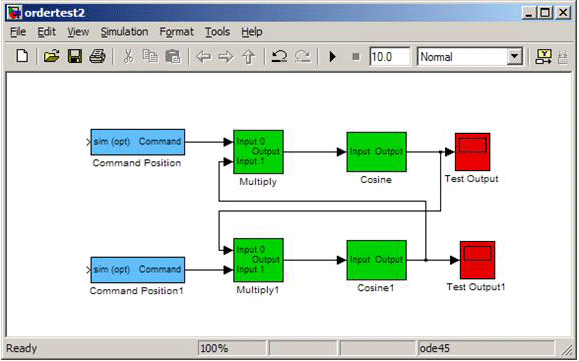The default algorithm orders the blocks as follows:

1. Command Position
2. Command Position1
3. Multiply
4. Cosine
5. Multiply1
6. Test Output
7. Cosine1
8. Test Output1

Note the Multiply block uses the output of Cosine1 from the previous sample. Suppose instead that you want Multiply 1 to execute first, using the output of Cosine from the previous sample. To ensure that Cosine 1 executes before Multiply, set each of their User Defined Block Priority fields.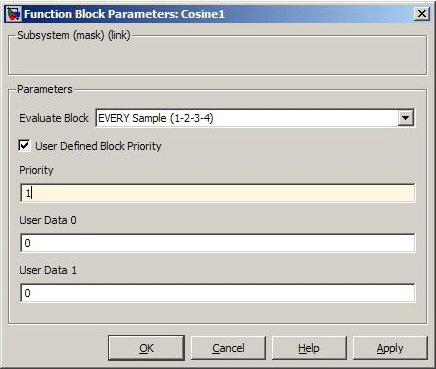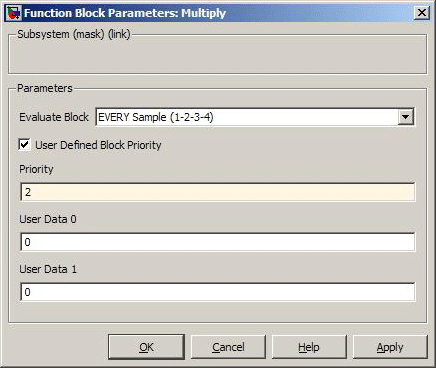Because all blocks with Priority 1 are executed before Priority 2, the final ordering is:

1. Command Position
2. Command Position1
3. Multiply1
4. Cosine1
5. Multiply
6. est Output1
7. Cosine
8. Test Output

Subsystems

Subsystems are scheduled as atomic units. All blocks in a Subsytem are executed sequentially, with no intervening blocks from outside the Subsystem. User Defined Block Priority for blocks in Subsystems are only used for scheduling relative to other blocks in the same Subsystem.

 Legal Notice  |  Tech Email  |  Feedback        Copyright © 2001-2009 Motion Engineering